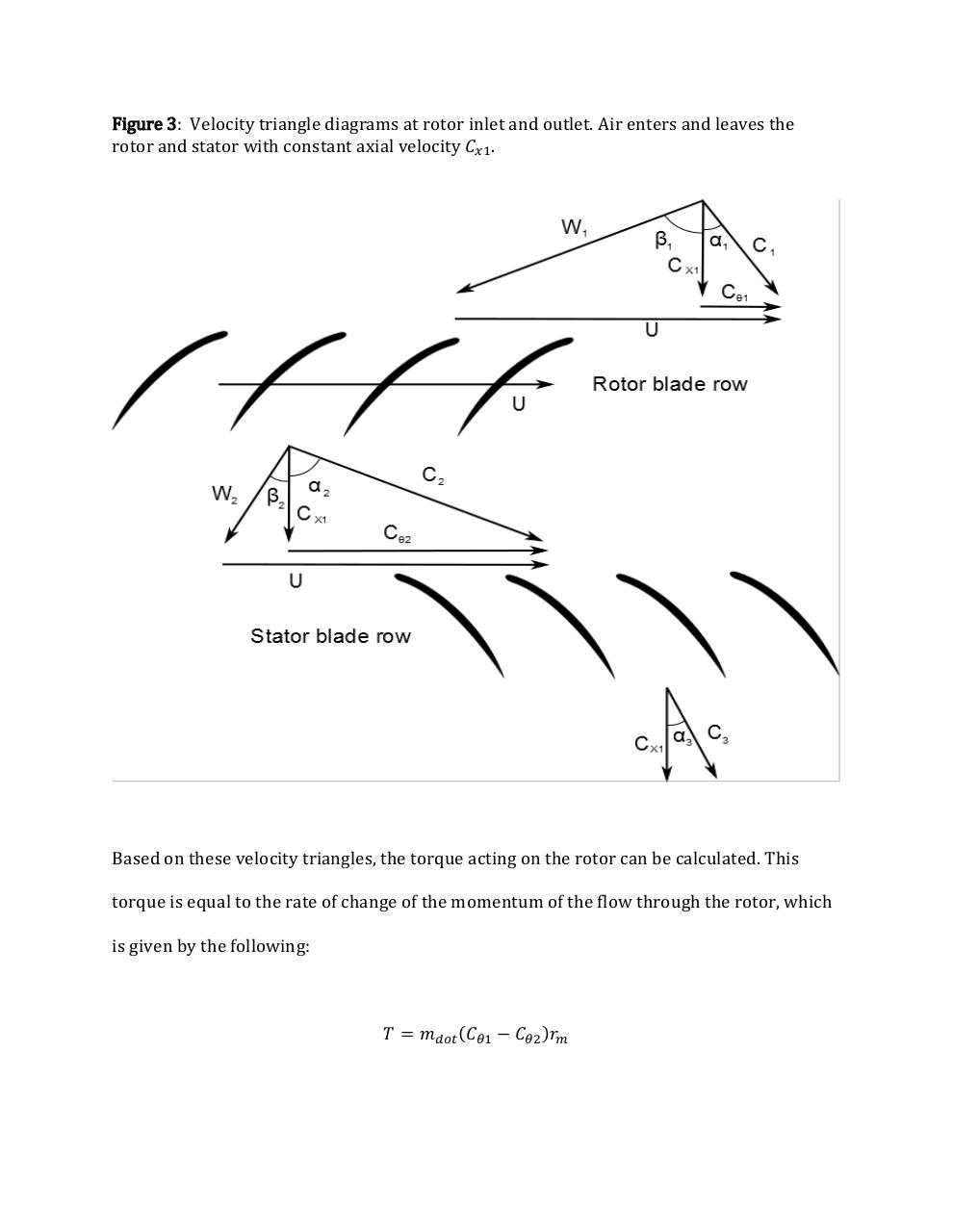# midterm comp.pdfPage 1 2 3 4 5 6

#### Text preview

Figure 3: Velocity triangle diagrams at rotor inlet and outlet. Air enters and leaves the
rotor and stator with constant axial velocity 𝐶𝑥1 .

Based on these velocity triangles, the torque acting on the rotor can be calculated. This
torque is equal to the rate of change of the momentum of the flow through the rotor, which
is given by the following:

𝑇 = 𝑚𝑑𝑜𝑡 (𝐶𝜃1 − 𝐶𝜃2 )𝑟𝑚